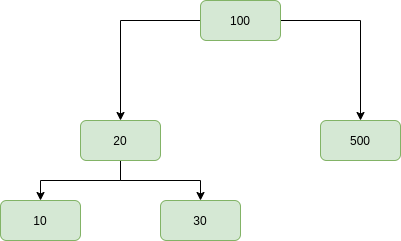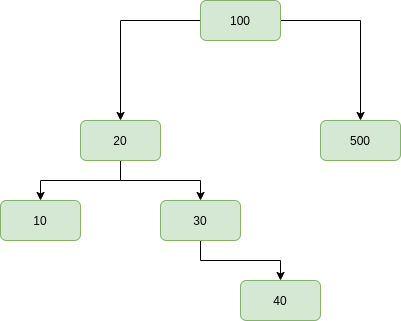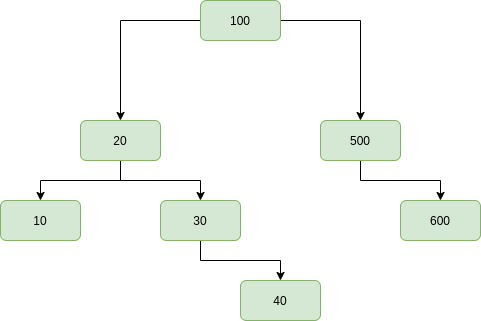# Insert a node in Binary Search Tree Iteratively

• Difficulty Level : Basic
• Last Updated : 20 Jul, 2022

A recursive approach to insert a new node in a BST is already discussed in the post: Binary Search Tree | SET 1. In this post, an iterative approach to insert a node in BST is discussed.

Insertion of a Key

A new key is always inserted at the leaf node. Start searching a key from root till we hit a leaf node. Once a leaf node is found, the new node is added as a child of the leaf node.
Example

Input:To the given BST insert 40Output:Explanation:The new node 40 is a leaf node. Start searching from the root till a leaf node is hit, i.e while searching if a new value is greater than current node move to right child else to left child.
Input:To the given BST insert 600Output:Explanation:The new node 600 is a leaf node. Start searching from the root till a leaf node is hit, i.e while searching if a new value is greater than current node move to right child else to left child.

Solution:
Approach

• It is to be noted that new keys are always inserted at the leaf node.
• Start from root and run a loop until a null pointer is reached.
• Keep the previous pointer of the current node stored.
• If the current node is null then create and insert the new node there and make it as one of the children of the parent/previous node depending on its value.
• If the value of current node is less than the new value then move to the right child of current node else move to the left child.

Below is the implementation of the above approach:

## C++

 `// C++ program to demonstrate insert operation``// in binary search tree``#include ``using` `namespace` `std;` `// BST node``struct` `Node {``    ``int` `key;``    ``struct` `Node *left, *right;``};` `// Utility function to create a new node``Node* newNode(``int` `data)``{``    ``Node* temp = ``new` `Node;` `    ``temp->key = data;` `    ``temp->left = NULL;``    ``temp->right = NULL;` `    ``return` `temp;``}` `// A utility function to insert a new``// Node with given key in BST``Node* insert(Node* root, ``int` `key)``{``    ``// Create a new Node containing``    ``// the new element``    ``Node* newnode = newNode(key);` `    ``// Pointer to start traversing from root and``    ``// traverses downward path to search``    ``// where the new node to be inserted``    ``Node* x = root;` `    ``// Pointer y maintains the trailing``    ``// pointer of x``    ``Node* y = NULL;` `    ``while` `(x != NULL) {``        ``y = x;``        ``if` `(key < x->key)``            ``x = x->left;``        ``else``            ``x = x->right;``    ``}` `    ``// If the root is NULL i.e the tree is empty``    ``// The new node is the root node``    ``if` `(y == NULL)``        ``y = newnode;` `    ``// If the new key is less than the leaf node key``    ``// Assign the new node to be its left child``    ``else` `if` `(key < y->key)``        ``y->left = newnode;` `    ``// else assign the new node its right child``    ``else``        ``y->right = newnode;` `    ``// Returns the pointer where the``    ``// new node is inserted``    ``return` `y;``}` `// A utility function to do inorder``// traversal of BST``void` `Inorder(Node* root)``{``    ``if` `(root == NULL)``        ``return``;``    ``else` `{``        ``Inorder(root->left);``        ``cout << root->key << ``" "``;``        ``Inorder(root->right);``    ``}``}` `// Driver code``int` `main()``{``    ``/* Let us create following BST``            ``50``          ``/   \``        ``30      70``        ``/ \   / \``       ``20 40 60 80 */` `    ``Node* root = NULL;``    ``root = insert(root, 50);``    ``insert(root, 30);``    ``insert(root, 20);``    ``insert(root, 40);``    ``insert(root, 70);``    ``insert(root, 60);``    ``insert(root, 80);` `    ``// Print inorder traversal of the BST``    ``Inorder(root);` `    ``return` `0;``}`

## Java

 `// Java program to demonstrate insert operation``// in binary search tree``import` `java.util.*;``class` `solution``{``  ` `// BST node``static` `class` `Node {``    ``int` `key;``     ``Node left, right;``};``  ` `// Utility function to create a new node``static` `Node newNode(``int` `data)``{``    ``Node temp = ``new` `Node();``  ` `    ``temp.key = data;``  ` `    ``temp.left = ``null``;``    ``temp.right = ``null``;``  ` `    ``return` `temp;``}``  ` `// A utility function to insert a new``// Node with given key in BST``static` `Node insert(Node root, ``int` `key)``{``    ``// Create a new Node containing``    ``// the new element``    ``Node newnode = newNode(key);``  ` `    ``// Pointer to start traversing from root and``    ``// traverses downward path to search``    ``// where the new node to be inserted``    ``Node x = root;``  ` `    ``// Pointer y maintains the trailing``    ``// pointer of x``    ``Node y = ``null``;``  ` `    ``while` `(x != ``null``) {``        ``y = x;``        ``if` `(key < x.key)``            ``x = x.left;``        ``else``            ``x = x.right;``    ``}``  ` `    ``// If the root is null i.e the tree is empty``    ``// The new node is the root node``    ``if` `(y == ``null``)``        ``y = newnode;``  ` `    ``// If the new key is less than the leaf node key``    ``// Assign the new node to be its left child``    ``else` `if` `(key < y.key)``        ``y.left = newnode;``  ` `    ``// else assign the new node its right child``    ``else``        ``y.right = newnode;``  ` `    ``// Returns the pointer where the``    ``// new node is inserted``    ``return` `y;``}``  ` `// A utility function to do inorder``// traversal of BST``static` `void` `Inorder(Node root)``{``    ``if` `(root == ``null``)``        ``return``;``    ``else` `{``        ``Inorder(root.left);``        ``System.out.print( root.key +``" "``);``        ``Inorder(root.right);``    ``}``}``  ` `// Driver code``public` `static` `void` `main(String args[])``{``    ``/* Let us create following BST ``            ``50 ``          ``/   \ ``        ``30      70 ``        ``/ \   / \ ``       ``20 40 60 80 */``  ` `    ``Node root = ``null``;``    ``root = insert(root, ``50``);``    ``insert(root, ``30``);``    ``insert(root, ``20``);``    ``insert(root, ``40``);``    ``insert(root, ``70``);``    ``insert(root, ``60``);``    ``insert(root, ``80``);``  ` `    ``// Print inorder traversal of the BST``    ``Inorder(root);``  ` `}``}``//contributed by Arnab Kundu`

## Python3

 `"""Python3 program to demonstrate insert``operation in binary search tree """` `# A Binary Tree Node``# Utility function to create a``# new tree node``class` `newNode:` `    ``# Constructor to create a newNode``    ``def` `__init__(``self``, data):``        ``self``.key``=` `data``        ``self``.left ``=` `None``        ``self``.right ``=` `self``.parent ``=` `None` `# A utility function to insert a new``# Node with given key in BST``def` `insert(root, key):` `    ``# Create a new Node containing``    ``# the new element``    ``newnode ``=` `newNode(key)` `    ``# Pointer to start traversing from root``    ``# and traverses downward path to search``    ``# where the new node to be inserted``    ``x ``=` `root` `    ``# Pointer y maintains the trailing``    ``# pointer of x``    ``y ``=` `None` `    ``while` `(x !``=` `None``):``        ``y ``=` `x``        ``if` `(key < x.key):``            ``x ``=` `x.left``        ``else``:``            ``x ``=` `x.right``    ` `    ``# If the root is None i.e the tree is``    ``# empty. The new node is the root node``    ``if` `(y ``=``=` `None``):``        ``y ``=` `newnode` `    ``# If the new key is less than the leaf node key``    ``# Assign the new node to be its left child``    ``elif` `(key < y.key):``        ``y.left ``=` `newnode` `    ``# else assign the new node its``    ``# right child``    ``else``:``        ``y.right ``=` `newnode` `    ``# Returns the pointer where the``    ``# new node is inserted``    ``return` `y` `# A utility function to do inorder``# traversal of BST``def` `Inorder(root) :` `    ``if` `(root ``=``=` `None``) :``        ``return``    ``else``:``        ``Inorder(root.left)``        ``print``( root.key, end ``=` `" "` `)``        ``Inorder(root.right)``                        ` `# Driver Code``if` `__name__ ``=``=` `'__main__'``:` `    ``""" Let us create following BST``            ``50``        ``/ \``        ``30     70``        ``/ \ / \``    ``20 40 60 80 """` `    ``root ``=` `None``    ``root ``=` `insert(root, ``50``)``    ``insert(root, ``30``)``    ``insert(root, ``20``)``    ``insert(root, ``40``)``    ``insert(root, ``70``)``    ``insert(root, ``60``)``    ``insert(root, ``80``)` `    ``# Pr inorder traversal of the BST``    ``Inorder(root)` `# This code is contributed by``# SHUBHAMSINGH10`

## C#

 `// C# program to demonstrate insert ``// operation in binary search tree``using` `System;` `class` `GFG``{``    ``// BST node``    ``class` `Node``    ``{``        ``public` `int` `key;``        ``public` `Node left, right;``    ``};` `    ``// Utility function to create a new node``    ``static` `Node newNode(``int` `data)``    ``{``        ``Node temp = ``new` `Node();` `        ``temp.key = data;` `        ``temp.left = ``null``;``        ``temp.right = ``null``;` `        ``return` `temp;``    ``}` `    ``// A utility function to insert a new``    ``// Node with given key in BST``    ``static` `Node insert(Node root, ``int` `key)``    ``{``        ``// Create a new Node containing``        ``// the new element``        ``Node newnode = newNode(key);` `        ``// Pointer to start traversing from root and``        ``// traverses downward path to search``        ``// where the new node to be inserted``        ``Node x = root;` `        ``// Pointer y maintains the trailing``        ``// pointer of x``        ``Node y = ``null``;` `        ``while` `(x != ``null``)``        ``{``            ``y = x;``            ``if` `(key < x.key)``                ``x = x.left;``            ``else``                ``x = x.right;``        ``}` `        ``// If the root is null i.e the tree is empty``        ``// The new node is the root node``        ``if` `(y == ``null``)``            ``y = newnode;` `        ``// If the new key is less than the leaf node key``        ``// Assign the new node to be its left child``        ``else` `if` `(key < y.key)``            ``y.left = newnode;` `        ``// else assign the new node its right child``        ``else``            ``y.right = newnode;` `        ``// Returns the pointer where the``        ``// new node is inserted``        ``return` `y;``    ``}` `    ``// A utility function to do inorder``    ``// traversal of BST``    ``static` `void` `Inorder(Node root)``    ``{``        ``if` `(root == ``null``)``            ``return``;``        ``else``        ``{``            ``Inorder(root.left);``            ``Console.Write( root.key +``" "``);``            ``Inorder(root.right);``        ``}``    ``}` `    ``// Driver code``    ``public` `static` `void` `Main(String []args)``    ``{``        ``/* Let us create following BST``                ``50``            ``/ \``            ``30 70``            ``/ \ / \``        ``20 40 60 80 */` `        ``Node root = ``null``;``        ``root = insert(root, 50);``        ``insert(root, 30);``        ``insert(root, 20);``        ``insert(root, 40);``        ``insert(root, 70);``        ``insert(root, 60);``        ``insert(root, 80);` `        ``// Print inorder traversal of the BST``        ``Inorder(root);``    ``}``}` `// This code is contributed 29AjayKumar`

## Javascript

 ``

Output:

`20 30 40 50 60 70 80`

Complexity Analysis:

• Time Complexity : O(h), where h is height of binary search tree. In worst case the height is equal to number of nodes.

• Space Complexity: O(1), no extra space is required.

My Personal Notes arrow_drop_up